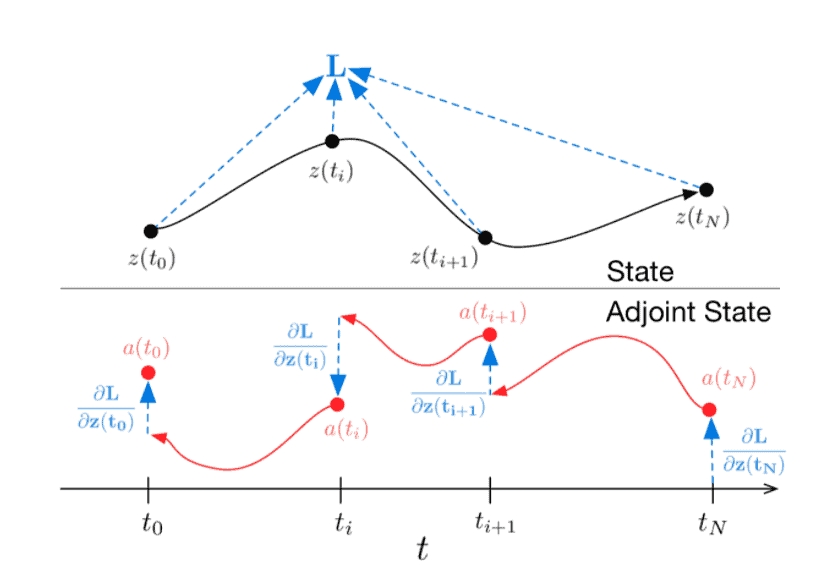# AMATH 342/CM 352: Computational Methods for Differential Equations

Offered every fall and spring term.

## Brief description:

Mathematical models based on Ordinary Differential Equations (ODEs) are ubiquitous these days, arising in all areas of science and engineering, and also in finance and economics. In complex models, the differential equations cannot be solved exactly, and one has to rely on approximate solutions obtained using numerical methods on computers.

The goal of this course is threefold. You will receive a solid introduction to the theory of numerical methods for differential equations (with derivations of the methods and some proofs). You will learn to implement the computational methods efficiently in Matlab, and you will apply the methods to problems in several fields, for example, climate modeling, combustion, control theory, and mathematical biology.

## Introduction by an example:Ordinary Differential Equations

Imagine a problem: there is a process following an unknown ODE and some (noisy) observations along its trajectory

$\frac{d z}{d t}=f(z(t), t)$
$\left{\left(z_{0}, t_{0}\right),\left(z_{1}, t_{1}\right), \ldots,\left(z_{M}, t_{M}\right)\right}$

Is it possible to find an approximation $\widehat{f}(z, t, \theta)$ of dynamics function $f(z, t) ?$
First, consider a somewhat simpler task: there are only 2 observations, at the beginning and at the end of the trajectory, $\left(z_{0}, t_{0}\right),\left(z_{1}, t_{1}\right)$. One starts the evolution of the system from $z_{0}, t_{0}$ for time $t_{1}-t_{0}$ with some parameterized dynamics function using any ODE initial value solver. After that, one ends up being at some new state $\hat{z_{1}}, t_{1},$ compares it with the observation $z_{1},$ and tries to minimize the difference by varying the parameters $\theta$.

Or, more formally, consider optimizing the following loss function $L\left(\hat{z}{1}\right):$ $$L\left(z\left(t{1}\right)\right)=L\left(\int_{t_{0}}^{t_{1}} f(z(t), t, \theta) d t\right)=L\left(\text { ODESolve }\left(z\left(t_{0}\right), f, t_{0}, t_{1}, \theta\right)\right)$$

In case you don’t want to dig into the maths, the above figure representes what is going on. Black trajectory represents solving the ODE during forward propagation. Red arrows represent solving the adjoint ODE during backpropagation.
To optimize $L$ one needs to compute the gradients wrt. its parameters: $z\left(t_{0}\right), t_{0}, t_{1}, \theta .$ To do this let us first determine how loss depends on the state at every moment of time $(z(t))$ :
$$a(t)=-\frac{\partial L}{\partial z(t)}$$
$a(t)$ is called adjoint, its dynamics is given by another ODE, which can be thought of as an instantaneous analog of the chain rule
$$\frac{d a(t)}{d t}=-a(t) \frac{\partial f(z(t), t, \theta)}{\partial z}$$
Actual derivation of this particular formula can be found in the appendix of the original paper.
All vectors here are considered row vectors, whereas the original paper uses both column and row representations.
One can then compute
$$\frac{\partial L}{\partial z\left(t_{0}\right)}=\int_{t_{1}}^{t_{0}} a(t) \frac{\partial f(z(t), t, \theta)}{\partial z} d t$$

## Prerequisites:

AMATH 242 / CS 371 / CM 271, or permission of the instructor.

If you have completed your 2B term, and have good grades in AMATH 250, MATH 237/247 and MATH 235/245, the instructor will sign you into AMATH 342. The course includes an optional tutorial in Matlab which will ensure that the students who have not taken AMATH 242 are prepared for AMATH 342.

## Intended audience:

This course will be of interest to anyone who wants to be able to use computers to analyze mathematical models based on differential equations.

# AMATH 342/CM 352代写答疑辅导，请认准UpriviateTA拓扑，topology代写请认准UpriviateTA